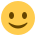#### Howdy, Stranger!

It looks like you're new here. If you want to get involved, click one of these buttons!

Supported by

# Button can't be pressed

edited January 2019

Hi,

I ran across your team's poster at Psychonomic Society and considering converting to your plugin. By the way, mousetrap is great! Thanks guys!

Anyway, I've been trying to make an experiment with the plugin and ran into a problem. That is, my design has four response alternatives, meaning four buttons. However, the fourth button can't be clicked and does not lead to the next trial. I've checked the code of it, but it does not seems to differ with codes associated with other buttons.

What am I doing wrong? I am attaching the code for the main display below.

Hansol

1. code for stimulus presentation
set duration 0
set description "Displays stimuli"
draw rect color=black fill=0 h=128 name="[tar4]" penwidth=1 show_if=always w=-192 x=512 y=-288 z_index=0
draw rect color=black fill=0 h=128 penwidth=1 show_if=always w=192 x=32 y=-384 z_index=0
draw rect color=black fill=0 h=128 penwidth=1 show_if=always w=192 x=-224 y=-384 z_index=0
draw rect color=black fill=0 h=128 penwidth=1 show_if=always w=192 x=-512 y=-288 z_index=0
draw textline center=1 color=black font_bold=no font_family=sans font_italic=no font_size=18 html=yes show_if=always text="[tar1]" x=-416 y=-224 z_index=0
draw textline center=1 color=black font_bold=no font_family=sans font_italic=no font_size=18 html=yes show_if=always text="[tar2]" x=-128 y=-320 z_index=0
draw textline center=1 color=black font_bold=no font_family=sans font_italic=no font_size=18 html=yes show_if=always text="[tar3]" x=128 y=-320 z_index=0
draw textline center=1 color=black font_bold=no font_family=sans font_italic=no font_size=18 html=yes show_if=always text="[tar4]" x=416 y=-224 z_index=0
draw textline center=1 color=black font_bold=no font_family=sans font_italic=no font_size=70 html=yes show_if=always text="+" x=0 y=-32 z_index=0
draw image center=1 file="[img1]" scale=0.7 show_if=always x=-192 y=288 z_index=0
draw image center=1 file="[img2]" scale=0.7 show_if=always x=192 y=288 z_index=0

1. code for "get_response"
warning_message
draw textline text="Please start moving" x=0 y=0
end
set update_feedback yes
set timeout infinite
set start_coordinates "0;280"
set skip_item no
set save_trajectories yes
set reset_mouse yes
set number_of_buttons 4
set mouse_buttons_allowed "left_button;right_button"
set max_initiation_time 1000
set logging_resolution 10
set description "Tracks mouse movements"
set correct_button "[correct]"
set click_required yes
set check_initiation_time no
set button4 "x=512 y=-288 w=192 h=128 name=[tar4]"
set button3 "x=32 y=-384 w=192 h=128 name=[tar3]"
set button2 "x=-224 y=-384 w=192 h=128 name=[tar2]"
set button1 "x=-512 y=-288 w=192 h=128 name=[tar1]"
set boundaries "upper=no lower=no left=no right=no"

• Hi Hansol,

thanks, great to hear you like it.

In the development version of mousetrap that you are using, there are two ways how to define buttons and you are kind of mixing the two of them. I would suggest to use the first variant (defining buttons using button names ).

For this, you would have to give a name tag to all four rect elements on the sketchpad:

``````draw rect color=black fill=0 h=128 name="[tar4]" penwidth=1 show_if=always w=-192 x=512 y=-288 z_index=0
draw rect color=black fill=0 h=128 name="[tar3]" penwidth=1 show_if=always w=192 x=32 y=-384 z_index=0
draw rect color=black fill=0 h=128 name="[tar2]" penwidth=1 show_if=always w=192 x=-224 y=-384 z_index=0
draw rect color=black fill=0 h=128 name="[tar1]" penwidth=1 show_if=always w=192 x=-512 y=-288 z_index=0
``````

Then you can simpliy the definition of the buttons in the mousetrap response item to:

``````set button4 "[tar4]"
set button3 "[tar3]"
set button2 "[tar2]"
set button1 "[tar1]"
``````

Now it should work.

By the way: I would suggest to change the name for correct variable from `correct` to something else, e.g., `correct_answer`, as `correct` is an OpenSesame variable that is created automatically to code whether the actual response matches the correct response.

Hope that helps!

Best,

Pascal

• Hi Pascal,

Thank you for your quick feedback. I did as you advised, but error occurs with this message
"The experiment did not finish normally for the following reason: Button1 is not specified correctly."

This was the first reason why I entered set button4 "x=512 y=-288 w=192 h=128 name=[tar4]" instead of just entering set button4 "[tar4]"..
Any ideas on why this might be happening?

Hansol

I tried defining buttons by their coordinates too. And it still wouldn't allow the fourth button to be clicked.

• Hi Pascal,

I figured out the issue here. It seems that there is a glitch in the program (either in the OpenSesame or the plugin).

from the code I initially posted, you can see that the width of the fourth button is "-192"

draw rect color=black fill=0 h=128 name="[tar4]" penwidth=1 show_if=always w=-192 x=512 y=-288

This was what made the button unclickable. This is weird, and I called it a glitch because the fourth button looks normal in the GUI display.

Anyway, thank you for your help!

Hansol